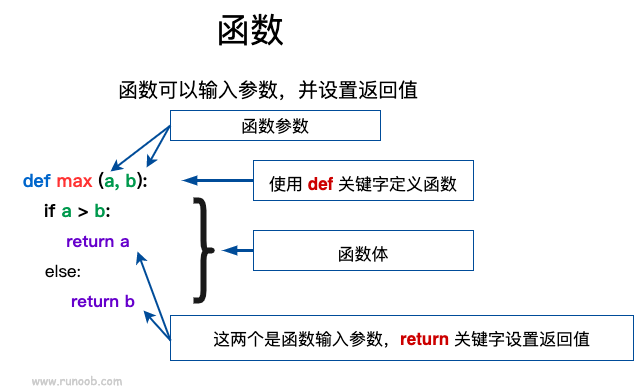# Python3基础之Python3函数

Python3.X作者：顾小北日期：2021-04-28点击：536

## 定义一个函数

• 函数代码块以 def 关键词开头，后接函数标识符名称和圆括号 ()
• 任何传入参数和自变量必须放在圆括号中间，圆括号之间可以用于定义参数。
• 函数的第一行语句可以选择性地使用文档字符串—用于存放函数说明。
• 函数内容以冒号 : 起始，并且缩进。
• return [表达式] 结束函数，选择性地返回一个值给调用方，不带表达式的 return 相当于返回 None。### 语法

Python 定义函数使用 def 关键字，一般格式如下：

```def 函数名（参数列表）:
函数体
```

### 实例

#!/usr/bin/python3

def hello() :
print("Hello World!")

hello()

## 实例(Python 3.0+)

#!/usr/bin/python3 def max(a, b): if a > b: return a else: return b a = 4 b = 5 print(max(a, b))

```5
```

## 实例(Python 3.0+)

#!/usr/bin/python3 # 计算面积函数 def area(width, height): return width * height def print_welcome(name): print("Welcome", name) print_welcome("Runoob") w = 4 h = 5 print("width =", w, " height =", h, " area =", area(w, h))

```Welcome Runoob
width = 4  height = 5  area = 20
```

## 实例(Python 3.0+)

#!/usr/bin/python3 # 定义函数 def printme( str ): # 打印任何传入的字符串 print (str) return # 调用函数 printme("我要调用用户自定义函数!") printme("再次调用同一函数")

```我要调用用户自定义函数!

```

## 参数传递

```a=[1,2,3]

a="Runoob"
```

### 可更改(mutable)与不可更改(immutable)对象

• 不可变类型：变量赋值 a=5 后再赋值 a=10，这里实际是新生成一个 int 值对象 10，再让 a 指向它，而 5 被丢弃，不是改变 a 的值，相当于新生成了 a。

• 可变类型：变量赋值 la=[1,2,3,4] 后再赋值 la=5 则是将 list la 的第三个元素值更改，本身la没有动，只是其内部的一部分值被修改了。

python 函数的参数传递：

• 不可变类型：类似 C++ 的值传递，如整数、字符串、元组。如 fun(a)，传递的只是 a 的值，没有影响 a 对象本身。如果在 fun(a) 内部修改 a 的值，则是新生成一个 a 的对象。

• 可变类型：类似 C++ 的引用传递，如 列表，字典。如 fun(la)，则是将 la 真正的传过去，修改后 fun 外部的 la 也会受影响

python 中一切都是对象，严格意义我们不能说值传递还是引用传递，我们应该说传不可变对象和传可变对象。

## 实例(Python 3.0+)

def change(a): print(id(a)) # 指向的是同一个对象 a=10 print(id(a)) # 一个新对象 a=1 print(id(a)) change(a)

```4379369136
4379369136
4379369424```

## 实例(Python 3.0+)

#!/usr/bin/python3 # 可写函数说明 def changeme( mylist ): "修改传入的列表" mylist.append([1,2,3,4]) print ("函数内取值: ", mylist) return # 调用changeme函数 mylist = [10,20,30] changeme( mylist ) print ("函数外取值: ", mylist)

```函数内取值:  [10, 20, 30, [1, 2, 3, 4]]

```

• 必需参数
• 关键字参数
• 默认参数
• 不定长参数

## 实例(Python 3.0+)

#!/usr/bin/python3 #可写函数说明 def printme( str ): "打印任何传入的字符串" print (str) return # 调用 printme 函数，不加参数会报错 printme()

```Traceback (most recent call last):
File "test.py", line 10, in <module>
printme()
TypeError: printme() missing 1 required positional argument: 'str'
```

## 实例(Python 3.0+)

#!/usr/bin/python3 #可写函数说明 def printme( str ): "打印任何传入的字符串" print (str) return #调用printme函数 printme( str = "菜鸟教程")

```菜鸟教程
```

## 实例(Python 3.0+)

#!/usr/bin/python3 #可写函数说明 def printinfo( name, age ): "打印任何传入的字符串" print ("名字: ", name) print ("年龄: ", age) return #调用printinfo函数 printinfo( age=50, name="runoob" )

```名字:  runoob

```

## 实例(Python 3.0+)

#!/usr/bin/python3 #可写函数说明 def printinfo( name, age = 35 ): "打印任何传入的字符串" print ("名字: ", name) print ("年龄: ", age) return #调用printinfo函数 printinfo( age=50, name="runoob" ) print ("------------------------") printinfo( name="runoob" )

```名字:  runoob

------------------------

```

### 不定长参数

```def functionname([formal_args,] *var_args_tuple ):
"函数_文档字符串"
function_suite
return [expression]
```

## 实例(Python 3.0+)

#!/usr/bin/python3 # 可写函数说明 def printinfo( arg1, *vartuple ): "打印任何传入的参数" print ("输出: ") print (arg1) print (vartuple) # 调用printinfo 函数 printinfo( 70, 60, 50 )

```输出:
70
(60, 50)```

## 实例(Python 3.0+)

#!/usr/bin/python3 # 可写函数说明 def printinfo( arg1, *vartuple ): "打印任何传入的参数" print ("输出: ") print (arg1) for var in vartuple: print (var) return # 调用printinfo 函数 printinfo( 10 ) printinfo( 70, 60, 50 )

```输出:
10

70
60
50
```

```def functionname([formal_args,] **var_args_dict ):
"函数_文档字符串"
function_suite
return [expression]
```

## 实例(Python 3.0+)

#!/usr/bin/python3 # 可写函数说明 def printinfo( arg1, **vardict ): "打印任何传入的参数" print ("输出: ") print (arg1) print (vardict) # 调用printinfo 函数 printinfo(1, a=2,b=3)

```输出:
1
{'a': 2, 'b': 3}
```

```def f(a,b,*,c):
return a+b+c```

```>>> def f(a,b,*,c):
...     return a+b+c
...
>>> f(1,2,3)   # 报错
Traceback (most recent call last):
File "<stdin>", line 1, in <module>
TypeError: f() takes 2 positional arguments but 3 were given
>>> f(1,2,c=3) # 正常
6
>>>```

## 匿名函数

python 使用 lambda 来创建匿名函数。

• lambda 只是一个表达式，函数体比 def 简单很多。
• lambda的主体是一个表达式，而不是一个代码块。仅仅能在lambda表达式中封装有限的逻辑进去。
• lambda 函数拥有自己的命名空间，且不能访问自己参数列表之外或全局命名空间里的参数。
• 虽然lambda函数看起来只能写一行，却不等同于C或C++的内联函数，后者的目的是调用小函数时不占用栈内存从而增加运行效率。

### 语法

lambda 函数的语法只包含一个语句，如下：

```lambda [arg1 [,arg2,.....argn]]:expression
```

## 实例(Python 3.0+)

#!/usr/bin/python3 # 可写函数说明 sum = lambda arg1, arg2: arg1 + arg2 # 调用sum函数 print ("相加后的值为 : ", sum( 10, 20 )) print ("相加后的值为 : ", sum( 20, 20 ))

```相加后的值为 :  30

```

## return语句

return [表达式] 语句用于退出函数，选择性地向调用方返回一个表达式。不带参数值的return语句返回None。之前的例子都没有示范如何返回数值，以下实例演示了 return 语句的用法：

## 实例(Python 3.0+)

#!/usr/bin/python3 # 可写函数说明 def sum( arg1, arg2 ): # 返回2个参数的和." total = arg1 + arg2 print ("函数内 : ", total) return total # 调用sum函数 total = sum( 10, 20 ) print ("函数外 : ", total)

```函数内 :  30

```

## 强制位置参数

Python3.8 新增了一个函数形参语法 / 用来指明函数形参必须使用指定位置参数，不能使用关键字参数的形式。

```def f(a, b, /, c, d, *, e, f):
print(a, b, c, d, e, f)```

`f(10, 20, 30, d=40, e=50, f=60)`

```f(10, b=20, c=30, d=40, e=50, f=60)   # b 不能使用关键字参数的形式
f(10, 20, 30, 40, 50, f=60)           # e 必须使用关键字参数的形式```